Algebra Commons™

1,047 Full-Text Articles 1,224 Authors 224,226 Downloads118 Institutions

All Articles in Algebra

1,047 full-text articles. Page 3 of 39.

Solvable Leibniz Superalgebras Whose Nilradical Is A Lie Superalgebra Of Maximal Nilindex, 2019National University of Uzbekistan; Institute of Mathematics, Uzbekistan Academy of Science

Solvable Leibniz Superalgebras Whose Nilradical Is A Lie Superalgebra Of Maximal Nilindex, Abror Khudoyberdiyev, Manuel Ladra, Khosiat Muratova

Bulletin of National University of Uzbekistan: Mathematics and Natural Sciences

In this paper, we investigate solvable Leibniz superalgebras whose nilradical is a Lie superalgebra with maximal nilindex.It should be noted that Lie superalgebra with a maximal nilindex only exists in the variety of Lie2,m when m is odd. We give the classification of all solvable Leibniz superalgebras such that even part is a Lie algebra and nilradical is a Lie superalgebra with a maximal index of nilpotency.

Monoidal Supercategories And Superadjunction, 2019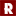University of Ottawa

Monoidal Supercategories And Superadjunction, Dene Lepine

Rose-Hulman Undergraduate Mathematics Journal

We define the notion of superadjunction in the context of supercategories. In particular, we give definitions in terms of counit-unit superadjunctions and hom-space superadjunctions, and prove that these two definitions are equivalent. These results generalize well-known statements in the non-super setting. In the super setting, they formalize some notions that have recently appeared in the literature. We conclude with a brief discussion of superadjunction in the language of string diagrams.

Strengthening Relationships Between Neural Ideals And Receptive Fields, 2019Texas A&M University

Strengthening Relationships Between Neural Ideals And Receptive Fields, Angelique Morvant

Rose-Hulman Undergraduate Mathematics Journal

Neural codes are collections of binary vectors that represent the firing patterns of neurons. The information given by a neural code C can be represented by its neural ideal JC. In turn, the polynomials in JC can be used to determine the relationships among the receptive fields of the neurons. In a paper by Curto et al., three such relationships, known as the Type 1-3 relations, were linked to the neural ideal by three if-and-only-if statements. Later, Garcia et al. discovered the Type 4-6 relations. These new relations differed from the first three in that they were related ...

Dissertation_Davis.Pdf, 2019University of Kentucky

Dissertation_Davis.Pdf, Brian Davis

brian davis

Simplices are the simplest" examples of polytopes, and yet they exhibit much of the rich and subtle combinatorics and commutative algebra of their more general cousins. In this way they are sufficiently complicated --- insights gained from their study can inform broader research in Ehrhart theory and associated fields.

In this dissertation we consider two previously unstudied properties of lattice simplices; one algebraic and one combinatorial. The first is the Poincare series of the associated semigroup algebra, which is substantially more complicated than the Hilbert series of that same algebra. The second is the partial ordering of the elements of the ...

Enhanced Koszulity In Galois Cohomology, 2019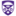The University of Western Ontario

Enhanced Koszulity In Galois Cohomology, Marina Palaisti

Electronic Thesis and Dissertation Repository

Despite their central role in Galois theory, absolute Galois groups remain rather mysterious; and one of the main problems of modern Galois theory is to characterize which profinite groups are realizable as absolute Galois groups over a prescribed field. Obtaining detailed knowledge of Galois cohomology is an important step to answering this problem. In our work we study various forms of enhanced Koszulity for quadratic algebras. Each has its own importance, but the common ground is that they all imply Koszulity. Applying this to Galois cohomology, we prove that, in all known cases of finitely generated pro-$p$-groups, Galois ...

Parametric Natura Morta, 2019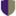Independent researcher, Palermo, Italy

Parametric Natura Morta, Maria C. Mannone

The STEAM Journal

Parametric equations can also be used to draw fruits, shells, and a cornucopia of a mathematical still life. Simple mathematics allows the creation of a variety of shapes and visual artworks, and it can also constitute a pedagogical tool for students.

Diagonal Sums Of Doubly Substochastic Matrices, 2019Georgian Court University

Diagonal Sums Of Doubly Substochastic Matrices, Lei Cao, Zhi Chen, Xuefeng Duan, Selcuk Koyuncu, Huilan Li

Let $\Omega_n$ denote the convex polytope of all $n\times n$ doubly stochastic matrices, and $\omega_{n}$ denote the convex polytope of all $n\times n$ doubly substochastic matrices. For a matrix $A\in\omega_n$, define the sub-defect of $A$ to be the smallest integer $k$ such that there exists an $(n+k)\times(n+k)$ doubly stochastic matrix containing $A$ as a submatrix. Let $\omega_{n,k}$ denote the subset of $\omega_n$ which contains all doubly substochastic matrices with sub-defect $k$. For $\pi$ a permutation of symmetric group of degree $n$, the sequence of elements $a_{1\pi(1 ... In-Sphere Property And Reverse Inequalities For Matrix Means, 2019Ton Duc Thang University In-Sphere Property And Reverse Inequalities For Matrix Means, Trung Hoa Dinh, Tin-Yau Tam, Bich Khue T Vo Electronic Journal of Linear Algebra The in-sphere property for matrix means is studied. It is proved that the matrix power mean satisfies in-sphere property with respect to the Hilbert-Schmidt norm. A new characterization of the matrix arithmetic mean is provided. Some reverse AGM inequalities involving unitarily invariant norms and operator monotone functions are also obtained. Surjective Additive Rank-1 Preservers On Hessenberg Matrices, 2019Walailak University Surjective Additive Rank-1 Preservers On Hessenberg Matrices, Prathomjit Khachorncharoenkul, Sajee Pianskool Electronic Journal of Linear Algebra Let$H_{n}(\mathbb{F})$be the space of all$n\times n$upper Hessenberg matrices over a field~$\mathbb{F}$, where$n$is a positive integer greater than two. In this paper, surjective additive maps preserving rank-$1$on$H_{n}(\mathbb{F})$are characterized. 2019Faculty of Sciences and Mathematics, University of Niš Solving The Sylvester Equation Ax-Xb=C When$\Sigma(A)\Cap\Sigma(B)\Neq\Emptyset$, Nebojša Č. Dinčić Electronic Journal of Linear Algebra The method for solving the Sylvester equation$AX-XB=C$in complex matrix case, when$\sigma(A)\cap\sigma(B)\neq \emptyset$, by using Jordan normal form is given. Also, the approach via Schur decomposition is presented. A Re-Emergent Analysis Of Early Algebraic Learning, 2019Portland State University A Re-Emergent Analysis Of Early Algebraic Learning, Steven Boyce, Diana Moss Extension Research In this paper, we discuss a novel approach for collaborative retrospective analysis. One researcher was directly involved in a classroom teaching experiment, adopting an emergent perspective as an interpreter-witness of classroom interactions during a four-week algebra instructional unit with sixth-grade students. The other researcher experienced and analyzed the data in reverse chronological order. We describe how this re-emergent perspective revealed aspects of students’ early algebraic reasoning. Resolution Of Conjectures Related To Lights Out! And Cartesian Products, 2019University of Wyoming Resolution Of Conjectures Related To Lights Out! And Cartesian Products, Bryan A. Curtis, Jonathan Earl, David Livingston, Bryan L. Shader Electronic Journal of Linear Algebra Lights Out!\ is a game played on a$5 \times 5$grid of lights, or more generally on a graph. Pressing lights on the grid allows the player to turn off neighboring lights. The goal of the game is to start with a given initial configuration of lit lights and reach a state where all lights are out. Two conjectures posed in a recently published paper about Lights Out!\ on Cartesian products of graphs are resolved. A Note On Linear Preservers Of Semipositive And Minimally Semipositive Matrices, 2019Indian Institute of Science, Bengaluru A Note On Linear Preservers Of Semipositive And Minimally Semipositive Matrices, Projesh Nath Choudhury, Rajesh Kannan, K. C. Sivakumar Electronic Journal of Linear Algebra Semipositive matrices (matrices that map at least one nonnegative vector to a positive vector) and minimally semipositive matrices (semipositive matrices whose no column-deleted submatrix is semipositive) are well studied in matrix theory. In this short note, the structure of linear maps which preserve the set of all semipositive/minimally semipositive matrices is studied. An open problem is solved, and some ambiguities in the article [J. Dorsey, T. Gannon, N. Jacobson, C.R. Johnson and M. Turnansky. Linear preservers of semi-positive matrices. {\em Linear and Multilinear Algebra}, 64:1853--1862, 2016.] are clarified. Vector Cross Product Differential And Difference Equations In R^3 And In R^7, 2019University of Beira Interior Vector Cross Product Differential And Difference Equations In R^3 And In R^7, Patrícia D. Beites, Alejandro P. Nicolás, Paulo Saraiva, José Vitória Electronic Journal of Linear Algebra Through a matrix approach of the$2$-fold vector cross product in$\mathbb{R}^3$and in$\mathbb{R}^7$, some vector cross product differential and difference equations are studied. Either the classical theory or convenient Drazin inverses, of elements belonging to the class of index$1\$ matrices, are applied.

Equivalence Of Classical And Quantum Codes, 2019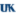University of Kentucky

Equivalence Of Classical And Quantum Codes, Tefjol Pllaha

Theses and Dissertations--Mathematics

In classical and quantum information theory there are different types of error-correcting codes being used. We study the equivalence of codes via a classification of their isometries. The isometries of various codes over Frobenius alphabets endowed with various weights typically have a rich and predictable structure. On the other hand, when the alphabet is not Frobenius the isometry group behaves unpredictably. We use character theory to develop a duality theory of partitions over Frobenius bimodules, which is then used to study the equivalence of codes. We also consider instances of codes over non-Frobenius alphabets and establish their isometry groups. Secondly ...

Upper Dimension And Bases Of Zero-Divisor Graphs Of Commutative Rings, 2019University of Kashmir, Srinagar, India

Upper Dimension And Bases Of Zero-Divisor Graphs Of Commutative Rings, S. Pirzada, M. Aijaza, Shane P. Redmond

EKU Faculty and Staff Scholarship

For a commutative ring R with non-zero zero divisor set Z∗(R), the zero divisor graph of R is Γ(R) with vertex set Z∗(R), where two distinct vertices x and y are adjacent if and only if x y = 0. The upper dimension and the resolving number of a zero divisor graph Γ(R) of some rings are determined. We provide certain classes of rings which have the same upper dimension and metric dimension and give an example of a ring for which these values do not coincide. Further, we obtain some bounds for the upper dimension in ...

An Invitation To Linear Algebra, 2019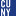CUNY Queensborough Community College

An Invitation To Linear Algebra, David N. Pham, Jonathon Funk, Wenjian Liu

Open Educational Resources

This is an OER textbook on linear algebra.

Lattice Simplices: Sufficiently Complicated, 2019University of Kentucky

Lattice Simplices: Sufficiently Complicated, Brian Davis

Theses and Dissertations--Mathematics

Simplices are the "simplest" examples of polytopes, and yet they exhibit much of the rich and subtle combinatorics and commutative algebra of their more general cousins. In this way they are sufficiently complicated --- insights gained from their study can inform broader research in Ehrhart theory and associated fields.

In this dissertation we consider two previously unstudied properties of lattice simplices; one algebraic and one combinatorial. The first is the Poincar\'e series of the associated semigroup algebra, which is substantially more complicated than the Hilbert series of that same algebra. The second is the partial ordering of the elements of ...

The State Of Lexicodes And Ferrers Diagram Rank-Metric Codes, 2019University of Kentucky

The State Of Lexicodes And Ferrers Diagram Rank-Metric Codes, Jared E. Antrobus

Theses and Dissertations--Mathematics

In coding theory we wish to find as many codewords as possible, while simultaneously maintaining high distance between codewords to ease the detection and correction of errors. For linear codes, this translates to finding high-dimensional subspaces of a given metric space, where the induced distance between vectors stays above a specified minimum. In this work I describe the recent advances of this problem in the contexts of lexicodes and Ferrers diagram rank-metric codes.

In the first chapter, we study lexicodes. For a ring R, we describe a lexicographic ordering of the left R-module Rn. With this ordering we ...

2019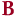Bard College

Polygonal Analogues To The Topological Tverberg And Van Kampen-Flores Theorems, Leah Leiner

Senior Projects Spring 2019

Tverberg’s theorem states that any set of (q-1)(d+1)+1 points in d-dimensional Euclidean space can be partitioned into q subsets whose convex hulls intersect. This is topologically equivalent to saying any continuous map from a (q-1)(d+1)-dimensional simplex to d-dimensional Euclidean space has q disjoint faces whose images intersect, given that q is a prime power. These continuous functions have a Fourier decomposition, which admits a Tverberg partition when all of the Fourier coefficients, except the constant coefficient, are zero. We have been working with continuous functions where all of the Fourier coefficients except the ...